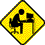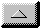Publications Department

Determination of G/T
by Richard Flagg, AH6NM (rf @ hawaii.rr.com)

Editor's Note: Ed Cole, The SETI League's volunteer Regional Coordinator for Alaska, has contributed a companion spreadsheet to perform the calculations discussed in this article.

G/T - A Receiving System Figure of Merit

The sensitivity of a radio telescope is a function of many factors including antenna gain (G) and system noise temperature (T). We all understand the need for high gain antennas and low noise preamplifiers. But how do we measure just how well the system is performing? A convenient figure of merit is the ratio (G/T) - the higher this ratio the better the sensitivity of the system to weak signals. To obtain G/T one could determine G and T separately, but these are difficult measurements. Fortunately it is relatively easy to obtain the ratio (G/T) by a single measurement (and a little arithmetic).

T - The Total System Noise Temperature

Before proceeding with the measurement of G/T let's discuss T in a little more detail. T is the total system noise temperature (in degrees Kelvin) and is equal to the sum of the noise generated in the receiving system (Tr) and the noise delivered from the antenna (Ta) when the antenna is looking at a region of the sky free of strong sources. Ta includes the galactic background temperature as well as additional noise picked up by the antenna sidelobes viewing the earth at ambient temperature.

The receiving system temperature (Tr) is related to the system noise factor (Fn) by:

Tr = (Fn - 1 )* 290 (Equation 1)

Where the noise factor (Fn) is simply the noise figure (NF) in dB expressed as a ratio:

Fn= (Log ^ -1) (NF / 10) (Equation 2)

Determining G/T

The principle behind determination of G/T is to measure the increase in noise power which occurs when the antenna is pointed first at a region of cold sky and then moved to a strong source of known flux density - usually the sun.

This ratio of received power is known as the Y-factor.

Y= Psun / Pcold sky (Equation 3)

The following equation shows the relationship between G/T, the measured Y-factor, and the value of solar flux (F) at the observing frequency.

G/T = (Y - 1) * 8 * pi * k * L / (F * Lam^2) (Equation 4)

where:

• Y = sun noise rise expressed as a ratio (not dB)
• k = Boltzmann's constant 1.38 *10^-23 joules/deg K
• L = beamsize correction factor
• Lam = wavelength in meters (at the operating frequency fo)
• F = solar flux at fo in watts / meter^2 / Hz

Beamsize Correction (L)

The beamsize correction factor (L) is dependent upon antenna beamwidth. and approaches unity for small dishes with beamwidths larger than a few degrees. If your dish has a beamwidth larger than 2 or 3 degrees just set L=1 and forget about equation (5).

L = 1 + 0.38 (Ws / Wa)^2 (Equation 5)

where:

• Ws = diameter of the radio sun in degrees at fo
• Wa = antenna 3 dB beamwidth at fo

The diameter of the radio sun (Ws) is frequency dependent. Assume a value of 0.5 degrees for frequencies above 3000 MHz, 0.6 degrees for 1420 MHz, and 0.7 degrees for 400 MHz.

Solar Flux Density (F)

The next term which we need to discuss is (F) - the solar flux density at the test frequency. The USAF Space Command runs a worldwide solar radio monitoring network with stations in Massachusetts, Hawaii, Australia, and Italy. These stations measure solar flux density at 245, 410, 610, 1415, 2695, 4995, 8800, and 15400 MHz. If you are lucky enough to be operating near one of these eight "standard" frequencies then all you have to do is use the reported flux density. However if you are operating - say midway - between two given frequencies then you will need to interpolate between flux densities at the lower and higher frequencies. The best interpolation scheme is to graph the flux density at several frequencies and use a curve fitting routine to determine the flux density at your operating frequency.

The solar flux density obtained from the USAF must be multiplied by 10^-22 in order to get the units correct for use in equation (4). In other words, if the 1415 MHz solar flux density is 98 *10^-22 watts/meter^2/Hz, the operator may simply state "the solar flux at 1415 Mhz is 98".

The solar flux at 2800 MHz (10.7 cm) is measured at the Dominion Radio Astronomy Observatory in Canada. This flux should only be used for G/T calculations if you are operating at or near 2,800 MHz.

G/T Sample Calculation

Assume that you have measured a sun noise rise of 9 dB using your 1420 MHz radio telescope. The solar flux density at the test frequency of 1415 MHz is reported to be 98.

First convert the sun noise rise in dB to a power ratio:

Y = Log-1(dB increase/10) = Log^-1(9/10) = 7.94

Determine the other factors:

fo = 1420 MHz, so
Lam = (300/1420) = 0.211m
and Lam^2 = 0.045 m^2
F = 98 *10^-22 w/m^2/Hz
L= 1
(since you know that your 3 meter dish has a beamwidth of about 5 degrees)

and finally solving for G/T:

G/T = (Y-1)*8*pi*k*L / (F*Lam^2)
= ((7.94-1)*8**3.14*1.38*10^-23) / (98*10^-22*0.045)
G/T = 5.5

or expressed in dB:

G/T (dB) = 10 Log(G/T) = 10Log(5.5) = 7.4 dB

Great - the G/T is 7.4 dB - so what? Should you be walking around with a silly grin - or slinking around looking for a rock to hide under? Well, for one thing this number is a reference point by which to judge the value of any future modifications to the system. To put it in perspective lets do the calculation in reverse and estimate what values of G/T and Y are expected given an antenna size (gain) and preamp noise temperature.

Assume that your 3 meter dish with an efficiency of 50% has a calculated gain of 30 dBi (power ratio of 1000) and that your preamp is advertised to have a noise temperature of 45 degrees K. Further assumptions include 10 deg K due to the galactic background, 25 deg K due to spillover, 30 deg K due to 0.5 dB of attenuation between the feed and the preamp and 5 deg K due to the receiver and cable following the preamp. Therefore the total receiving system temperature is estimated to be:

T = 45 + 25 + 30 + 10 +5 = 115 deg K.

The expected value of G/T is therefore ( 1000/115 ) = 8.7 = 9.4 dB

By the way, we can do this calculation by converting temperature into dB referenced to 1 deg K. and leaving the dish gain in dBi. Our temperature expressed in this way is

T dB = 10 Log (115/1) = 20.6
And G/T in dB is simply ( 30dB - 20.6dB) = 9.4 dB

So the expected value of G/T was 9.4 dB but we measured 7.4 dB. Why? A number of factors could be responsible, but the effect has been to either lower the dish gain or raise the system temperature from what was assumed. Its time to make sure the feed is focused and free of bird nests, and that no unexpected losses exist in the receiving system.

One final calculation shows what value of Y is expected given assumptions about antenna gain and system temperature.

Rewriting equation (4) and solving for Y yields:

Y = ((G/T)(F*Lam^2)/(8*pi*k*L)) +1 (Equation 6)

Remember to enter G/T as the ratio - not in dB.

Y = 12.1, or expressed in dB, Y = 10.8 dB

If our system was working exactly as expected a sun noise rise of 10.8 dB would have been measured - corresponding to a calculated G/T of 9.4 dB.

Measuring Y

The determination of G/T is completely dependent on an accurate measurement of Y. Perhaps the easiest measurement technique is to use a power meter (or a true RMS voltmeter) connected to the receiver IF . For this measurement to work the receiver must be operating in a linear region. If the receiver saturates when the antenna is pointed to the sun you are going to measure a dissapointing Y factor and spend lots of time trying to fix something that isn't broken. Of course the receiver AGC should be turned off. The Y factor is simply the change in meter reading on and off the sun. The accuracy of this method is dependent on the linearity of both the power meter and the receiver.

A better technique is to use a precision adjustable RF attenuator located between the preamp and the receiver. An RF power meter is connected to the receiver IF. Set the attenuator to 0 dB when the antenna is looking at the cold sky and adjust the receiver gain to get a convenient reference level on the power meter. Point the dish at the sun and crank in attenuation until the power meter once again reads the cold sky reference level. The Y factor is equal to the amount of attenuation needed to return the meter reading to the reference. This technique could also be used by measuring the DC output voltage from the receiver detector if a power meter is not available. Accuracy of the attenuator method depends on calibration of the attenuator - not receiver and power meter linearity.

Whatever technique you use - measure Y several times and take an average. The average of five measurements is probably adequate. Try and use solar flux measurements obtained about the same time your measurements were made for the calculation of G/T.

Where to get the Solar Flux

As mentioned earlier - the USAF operates a worldwide solar flux monitoring network. These data are disseminated thru NOAA's Space Environment Center - Space Weather Operations group in Boulder, Colorado (303 497-3171). The Space Environment Center also distributes the solar flux data for all eight frequencies via the world wide web. Set your brouser to:

gopher://solar.sec.noaa.gov:70/00/latest/curind

The 2,800 MHz flux from Canada is available at:

http://www.drao.nrc.ca/icarus/www/current/current.flx

The Learmonth, Australia eight frequency data may be found at:

And finally - for the most recent 45 days of solar flux measurements, see:

http://www.sec.noaa.gov/ftpdir/lists/radio/45day_rad.txt

Well, that's what I know about G/T - hope this information is helpful. Please let me know if you find any errors or know of improvements to these measurement techniques. I hope the formulas aren't too hard to interpret. Again - let me know if you have trouble with them.email theWebmaster | Home | General | Memb Svcs | Publications | Press | Technical | Internet | Index | entire website copyright © The SETI League, Inc.; Maintained by Microcomm this page last updated 15 July 2006Top of Page